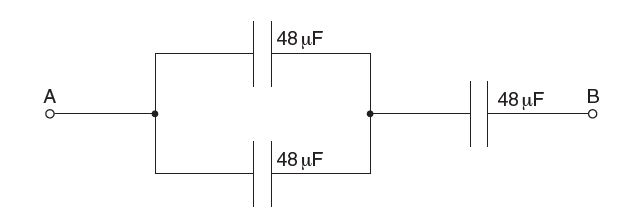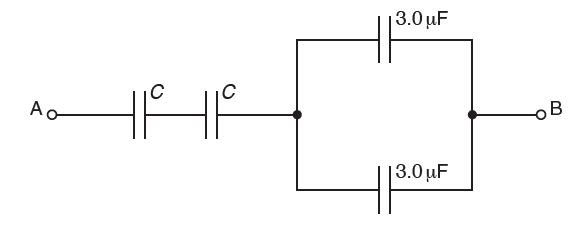Physic Tutorials

# How to solve questions on capacitance of capacitor for Cambridge A level and UTME

How to solve questions on capacitance of capacitor for Cambridge A level and UTME

Question 1

Three capacitors, each of capacitance 48 μF, are connected as shown in Fig below(a) Calculate the total capacitance between points A and B.

(b) The maximum safe potential difference that can be applied across any one capacitor is 6 V. Determine the maximum safe potential difference that can be applied between points A and B.{cambridge A level oct/nov 2014 p43}

solution

for parallel arrangement

c = c1 + c2

c = 48 + 48 = 96uf

96uf is in series with the third 48uf

1/c = 1/c1 + 1/c2

1/c = 1/96 + 1/48

1/c = 3/96

c = 32uf

(b)

In parallel same volatage across the capacitors, while in series same charge across the capacitors

The total charge flowing through the circuit is

C = Q/V

48 x 10^-6 = Q/6

Q = 48*6 x 10^-6 = 288 x 10^-6 c

p.d across the capacitor connected in parallel will be

C = Q/V

96 x 10^-6 = 288 x 10^-6 / V

v = 288 / 96 = 3v

the maximum safe potential difference that can be applied between points A and B = 3 + 6 =9v

or

p.d. across parallel combination is one half p.d. across single capacitor C1
total p.d. = 9 V

Question 2

The combined capacitance between terminals A and B of the arrangement shown in Fig below is 4.0 μF.Two capacitors each have capacitance C and the remaining capacitors each have capacitance 3.0 μF. The potential difference (p.d.) between terminals A and B is 12 V.
(i) Determine the capacitance C

(ii) Calculate the magnitude of the total positive charge transferred to the arrangement

(iii) Use your answer in (ii) to state the magnitude of the charge on one plate of
1. a capacitor of capacitance C,
2. a capacitor of capacitance 3.0 μF.{cambridge Alevel may/june p42}

solution

(i)

3.0 μF and 3.0 μF are in parallel

c= 3 + 3

c = 6μF

6μF is in series with c and c

1/Ct = 1/6 + 1/c + 1/c

1/4 = 1/6 + 1/c + 1/c

1/4 – 1/6 = 2/c

1/12 = 2/c

C =24uf

(ii)

The potential difference between terminal A and B is 12v

Total capacitance = total charge / total p.d

4 × 10^-6 = Q / 12

Q = 48 × 10^-6 c

(iii)

The charge on capacitor c is the same as the total charge in the arrangement = 48 × 10^-6 c

The charge on 3uf capacitor will be,

Firstly we need to know the p.d across the two capacitors arranged in parallel.

C = Q/v

6uf is the combined capacitance of the two capacitors

6 × 10^-6 = 48 × 10^-6 / v

V = 48/6 = 8v

The charge on the 3uf capacitor will be

3 × 10^-6 = Q/8

Cross multiply

Q = 24 × 10^-6 c

Question 3The diagram shows three capacitors C1, C2 and C3 of capacitances 2 μF, 6 μF and 3 μF respectively. The potential
differences across C1, C2 and C3 respectively are{UTME 2001}
A. 6V, 2V and 4V.
B. 6V, 4V and 2V.
C. 2V, 6V and 4V.
D. 4V, 6V and 2V.

Solution

The arrangement are in series, so the effective capacitance

1 / c = 1/ C1 + 1/ C2 + 1/ C3

1 / C = 1/ 2 + 1/6 + 1/ 3

1 / C = (3+1+2)/6

1/ C = 1/1

c = 1 μF

note that,

Q = CV

Q = 1*12 =12 C

For series arrangement the same charge will flow through the capacitors

on C1,

12 = 2V

V = 6v

on C2,

12 = 6V

V = 2 v

on C3,

12 = 3V

V = 4 v

The potential difference = 6v, 2v, 4v

A is the correct option

Question 4The diagram above shows two capacitors P and Q and capacitances 2μF and 4μF respectively connected to
a d.c. source. The ratio of energy stored in P to Q is{UTME 2001}
A. 1 : 2        B. 2 : 1         C. 4 : 1       D. 1 : 4

Solution

The arrangement is parallel, the implication of that is, the same voltage will be supplied across the capacitors

E = 1/2 CV^2

Ep = 1/2 *2*V^2 = V^2

Eq = 1/2*4*V^2 = 2 V^2

Their ratio = Ep /Eq = V^2 / 2V^2 = 1/2